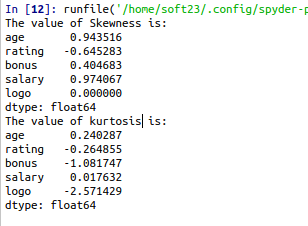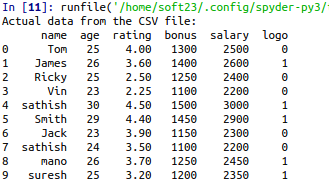• #5, First Floor, 4th Street Dr. Subbarayan Nagar Kodambakkam, Chennai-600 024 Landmark : Samiyar Madam
• pro@slogix.in
• +91- 81240 01111

### How to calculate Skewness and Kurtosis for a data set in python?

###### Description

To calculate the skewness and kurtosis for a sample data set using python.

#### Skewness:

It represents the shape of the distribution.

Skewness can be quantified to define the extent to which a distribution differs from a normal distribution.

For calculating skewness by using df.skew() python inbuilt function.

#### Kurtosis:

Kurtosis is the measure of thickness or heaviness of the given distribution.

Its actually represents the height of the distribution.

The distribution with kurtosis equal to3 is known as mesokurtic. A random variable which follows normal distribution has kurtosis 3.

If the kurtosis is less than three, the distribution is called as platykurtic. Here,the distribution has shorter and thinner tails than normal distribution.

If the kurtosis is greater than three, the distribution is called as leptykurtic. Here, the distribution has longer and fatter tails than normal distribution.

For calculating kurtosis by using df.kurtosis() python inbuilt function.

###### Sample Code

#import pandas library
import pandas as pd